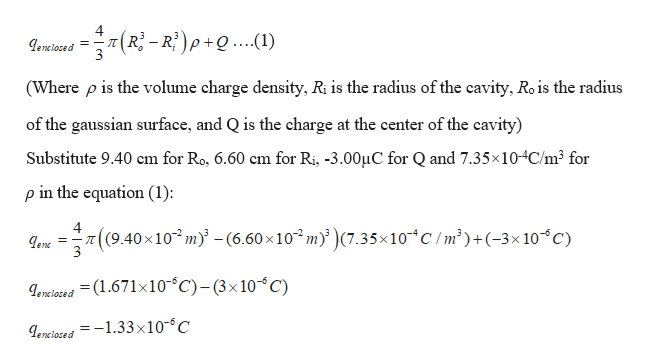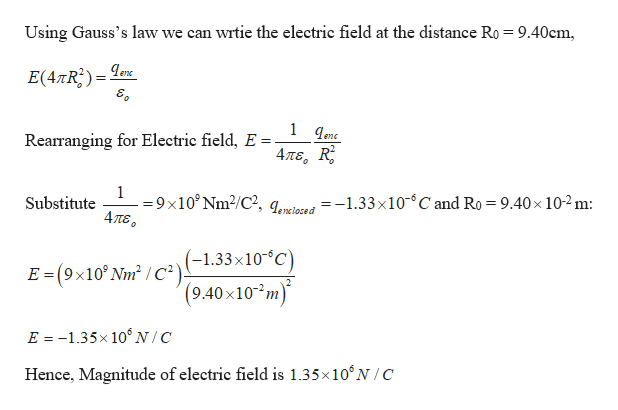# A point charge of -3.00 μC is located in the center of a spherical cavity of radius 6.60 cm inside an insulating spherical charged solid. The charge density in the solid is 7.35 × 10 −4 C/ m^3 .Part A Calculate the magnitude of the electric field inside the solid at a distance of 9.40 cm from the center of the cavity. Express your answer with the appropriate units. Part B Find the direction of this electric field.

Question
1 views

A point charge of -3.00 μC is located in the center of a spherical cavity of radius 6.60 cm inside an insulating spherical charged solid. The charge density in the solid is 7.35 × 10 −4 C/ m^3 .

Part A Calculate the magnitude of the electric field inside the solid at a distance of 9.40 cm from the center of the cavity. Express your answer with the appropriate units.

Part B Find the direction of this electric field.

check_circle

Step 1

Given:

Charge at the centre of the spherical cavity, Q = -3.00 µC

Radius of the spherical cavity, Ri = 6.60 cm = 6.60 ×10-2 m

Volume charge density of the spherical charged solid = 7.35×10-4 C/m3

Step 2

Consider a Gaussian sphere of radius R0 = 9.40cm = 9.40 ×10-2 m from the centre of the spherical cavity.

Write the expression for charge enclosed by the Gaussian sphere:help_outlineImage Transcriptionclose4 =-I genclosed 3 (Ri-Ri)p+Q..(1) (Where p is the volume charge density, Ri is the radius of the cavity, Ro is the radius of the gaussian surface, and Q is the charge at the center of the cavity) Substitute 9.40 cm for Ro, 6.60 cm for Ri, -3.00HC for Q and 7.35x10-4C/m3 for p in the equation (1) 4 ((9.40x10 m)-(6.60x10 m(7.35x10 C/m2)+(-3x 1oC) enc 3 enciosed (1.671x10 C)-(3x10C) Leneiaced =-1.33x10-C fullscreen
Step 3

(A) Calculate the electric field at the distance ...help_outlineImage TranscriptioncloseUsing Gauss's law we can wrtie the electric field at the distance Ro = 9.40cm, E(4TR2) enc 1 qenc Rearranging for Electric field, E Απε, R 1 9x10° Nm2/C?, qored=-1.33 x10 C and Ro = 9.40x 10-2m: 4πε. Substitute E (9x10 Nm2 /c(-1.33x10°C) (9.40 x10 m) E -1.35x 106N/C Hence, Magnitude of electric field is 1.35x10'N/C fullscreen

### Want to see the full answer?

See Solution

#### Want to see this answer and more?

Solutions are written by subject experts who are available 24/7. Questions are typically answered within 1 hour.*

See Solution
*Response times may vary by subject and question.
Tagged in

### Electric Charges and Fields